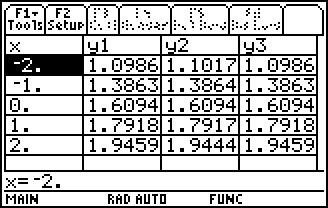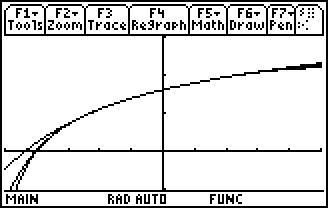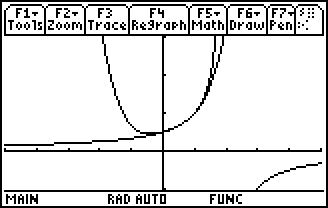# Activities

••• ##### Subject Area

• Math: Calculus: Series and Taylor Polynomials

• ##### Author9-12

45 Minutes

• ##### Device
• TI-89 / TI-89 Titanium

## Mr. Taylor, I Presume?#### Activity Overview

Explore Taylor polynomials graphically and analytically, as well as graphically determine the interval where the Taylor polynomial approximates the function it models.

#### Key Steps

•In this problem, students investigate a Taylor polynomial centered at zero.

Students will recall that this Taylor polynomial is also known as a Maclaurin polynomial. Students find the 4th degree Taylor polynomial that approximates f(x) = ln(x + 5) at x = 0. They will notice that the values are closest at the center and become farther apart the further the x-values are from the center.

•This problem also gives students the opportunity to explore different degrees of a Taylor polynomial. They use the Taylor command to find the respective polynomials and graph the function with each of the larger power polynomials in turn.

This will demonstrate that a Taylor polynomial will only approximate the function over a given interval, no matter how large the Taylor Polynomial is.

•At the end of this activity, students will be able to find the first few terms of a Taylor series approximation to a function for any given value of x. They will also be able to graph a function and its Taylor polynomials of various degrees to show their convergence to a function.